The FORECAST Procedure

Forecasting Methods

Subsections:

This section explains the forecasting methods used by PROC FORECAST.

STEPAR Method

In the STEPAR method, PROC FORECAST first fits a time trend model to the series and takes the difference between each value and the estimated trend. (This process is called detrending.) Then, the remaining variation is fit by using an autoregressive model.

The STEPAR method fits the autoregressive process to the residuals of the trend model by using a backwards-stepping method to select parameters. Because the trend and autoregressive parameters are fit in sequence rather than simultaneously, the parameter estimates are not optimal in a statistical sense. However, the estimates are usually close to optimal, and the method is computationally inexpensive.

The STEPAR Algorithm

The STEPAR method consists of the following computational steps:

1. Fit the trend model as specified by the TREND= option by using ordinary least-squares regression. This step detrends the data. The default trend model for the STEPAR method is TREND=2, a linear trend model.

2. Take the residuals from step 1 and compute the autocovariances to the number of lags specified by the NLAGS= option.

3. Regress the current values against the lags, using the autocovariances from step 2 in a Yule-Walker framework. Do not bring in any autoregressive parameter that is not significant at the level specified by the SLENTRY= option. (The default is SLENTRY=0.20.) Do not bring in any autoregressive parameter that results in a nonpositive-definite Toeplitz matrix.

4. Find the autoregressive parameter that is least significant. If the significance level is greater than the SLSTAY= value, remove the parameter from the model. (The default is SLSTAY=0.05.) Continue this process until only significant autoregressive parameters remain. If the OUTEST= option is specified, write the estimates to the OUTEST= data set.

5. Generate the forecasts by using the estimated model and output to the OUT= data set. Form the confidence limits by combining the trend variances with the autoregressive variances.

Missing values are tolerated in the series; the autocorrelations are estimated from the available data and tapered if necessary.

This method requires at least three passes through the data: two passes to fit the model and a third pass to initialize the autoregressive process and write to the output data set.

Default Value of the NLAGS= Option

If the NLAGS= option is not specified, the default value of the NLAGS= option is chosen based on the data frequency specified by the INTERVAL= option and on the number of observations in the input data set, if this can be determined in advance. (PROC FORECAST cannot determine the number of input observations before reading the data when a BY statement or a WHERE statement is used or if the data are from a tape format SAS data set or external database. The NLAGS= value must be fixed before the data are processed.)

If the INTERVAL= option is specified, the default NLAGS= value includes lags for up to three years plus one, subject to the maximum of 13 lags or one-third of the number of observations in your data set, whichever is less. If the number of observations in the input data set cannot be determined, the maximum NLAGS= default value is 13. If the INTERVAL= option is not specified, the default is NLAGS=13 or one-third the number of input observations, whichever is less.

If the Toeplitz matrix formed by the autocovariance matrix at a given step is not positive definite, the maximal number of autoregressive lags is reduced.

For example, for INTERVAL=QTR, the default is NLAGS=13 (that is,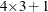) provided that there are at least 39 observations. The NLAGS= option default is always at least 3.

EXPO Method

Exponential smoothing is used when the METHOD=EXPO option is specified. The term exponential smoothing is derived from the computational scheme developed by Brown and others (Brown and Meyer, 1961; Brown, 1962). Estimates are computed with updating formulas that are developed across time series in a manner similar to smoothing.

The EXPO method fits a trend model such that the most recent data are weighted more heavily than data in the early part of the series. The weight of an observation is a geometric (exponential) function of the number of periods that the observation extends into the past relative to the current period. The weight function is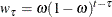whereis the observation number of the past observation, t is the current observation number, and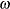is the weighting constant specified with the WEIGHT= option.

You specify the model with the TREND= option as follows:

• TREND=1 specifies single exponential smoothing (a constant model)

• TREND=2 specifies double exponential smoothing (a linear trend model)

• TREND=3 specifies triple exponential smoothing (a quadratic trend model)

Updating Equations

The single exponential smoothing operation is expressed by the formula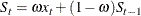where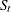is the smoothed value at the current period, t is the time index of the current period, and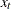is the current actual value of the series. The smoothed valueis the forecast of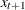and is calculated as the smoothing constanttimes the value of the series,, in the current period plus (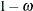) times the previous smoothed value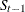, which is the forecast ofcomputed at time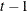.

Double and triple exponential smoothing are derived by applying exponential smoothing to the smoothed series, obtaining smoothed values as follows: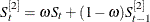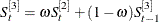Missing values after the start of the series are replaced with one-step-ahead predicted values, and the predicted value is then applied to the smoothing equations.

The polynomial time trend parameters CONSTANT, LINEAR, and QUAD in the OUTEST= data set are computed from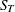,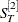, and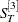, the final smoothed values at observation T, the last observation used to fit the model. In the OUTEST= data set, the values of,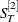, andare identified by _TYPE_=S1, _TYPE_=S2, and _TYPE_=S3, respectively.

Smoothing Weights

Exponential smoothing forecasts are forecasts for an integrated moving-average process; however, the weighting parameter is specified by the user rather than estimated from the data. Experience has shown that good values for the WEIGHT= option are between 0.05 and 0.3. As a general rule, smaller smoothing weights are appropriate for series with a slowly changing trend, while larger weights are appropriate for volatile series with a rapidly changing trend. If unspecified, the weight defaults to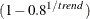, where trend is the value of the TREND= option. This produces defaults of WEIGHT=0.2 for TREND=1, WEIGHT=0.10557 for TREND=2, and WEIGHT=0.07168 for TREND=3.

The ESM procedure can be used to forecast time series by using exponential smoothing with smoothing weights that are optimized automatically. See Chapter 14: The ESM Procedure.

The Time Series Forecasting System provides for exponential smoothing models and enables you to either specify or optimize the smoothing weights. See Chapter 46: Getting Started with Time Series Forecasting, for details.

Confidence Limits

The confidence limits for exponential smoothing forecasts are calculated as they would be for an exponentially weighted time trend regression, using the simplifying assumption of an infinite number of observations. The variance estimate is computed by using the mean square of the unweighted one-step-ahead forecast residuals.

More detailed descriptions of the forecast computations can be found in Montgomery and Johnson (1976); Brown (1962).

WINTERS Method

The WINTERS method uses updating equations similar to exponential smoothing to fit parameters for the model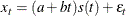where a and b are the trend parameters and the function s (t ) selects the seasonal parameter for the season that corresponds to time t.

The WINTERS method assumes that the series values are positive. If negative or zero values are found in the series, a warning is printed and the values are treated as missing.

The preceding standard WINTERS model uses a linear trend. However, PROC FORECAST can also fit a version of the WINTERS method that uses a quadratic trend. When TREND=3 is specified for METHOD=WINTERS, PROC FORECAST fits the following model: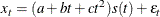The quadratic trend version of the Winters method is often unstable, and its use is not recommended.

When TREND=1 is specified, the following constant trend version is fit: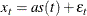The default for the WINTERS method is TREND=2, which produces the standard linear trend model.

Seasonal Factors

The notation s (t) represents the selection of the seasonal factor used for different time periods. For example, if INTERVAL=DAY and SEASONS=MONTH, there are 12 seasonal factors, one for each month in the year, and the time index t is measured in days. For any observation, t is determined by the ID variable and s (t) selects the seasonal factor for the month that t falls in. For example, if t is 9 February 1993 then s (t) is the seasonal parameter for February.

When there are multiple seasons specified, s (t) is the product of the parameters for the seasons. For example, if SEASONS=(MONTH DAY), then s (t) is the product of the seasonal parameter for the month that corresponds to the period t and the seasonal parameter for the day of the week that corresponds to period t. When the SEASONS= option is not specified, the seasonal factors s (t) are not included in the model. See the section Specifying Seasonality for more information about specifying multiple seasonal factors.

Updating Equations

This section shows the updating equations for the Winters method. In the following formula,is the actual value of the series at time t;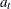is the smoothed value of the series at time t;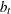is the smoothed trend at time t;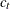is the smoothed quadratic trend at time t;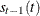selects the old value of the seasonal factor that corresponds to time t before the seasonal factors are updated.

The estimates of the constant, linear, and quadratic trend parameters are updated by using the following equations:

For TREND=3,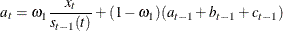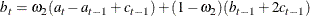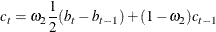For TREND=2,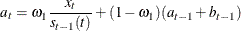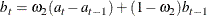For TREND=1,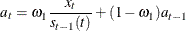In this updating system, the trend polynomial is always centered at the current period so that the intercept parameter of the trend polynomial for predicted values at times after t is always the updated intercept parameter. The predicted value forperiods ahead is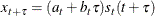The seasonal parameters are updated when the season changes in the data, using the mean of the ratios of the actual to the predicted values for the season. For example, if SEASONS=MONTH and INTERVAL=DAY, then when the observation for the first of February is encountered, the seasonal parameter for January is updated by using the formula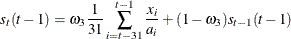where t is February 1 of the current year,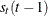is the seasonal parameter for January updated with the data available at time t, and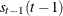is the seasonal parameter for January of the previous year.

When multiple seasons are used,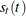is a product of seasonal factors. For example, if SEASONS=(MONTH DAY) thenis the product of the seasonal factors for the month and for the day of the week: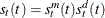.

The factor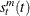is updated at the start of each month by using a modification of the preceding formula that adjusts for the presence of the other seasonal by dividing the summands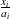by the that corresponds to day of the week effect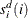.

Similarly, the factor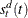is updated by using the following formula: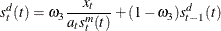where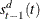is the seasonal factor for the same day of the previous week.

Missing values after the start of the series are replaced with one-step-ahead predicted values, and the predicted value is substituted for xand applied to the updating equations.

Normalization

The parameters are normalized so that the seasonal factors for each cycle have a mean of 1.0. This normalization is performed after each complete cycle and at the end of the data. Thus, if INTERVAL=MONTH and SEASONS=MONTH are specified and a series begins with a July value, then the seasonal factors for the series are normalized at each observation for July and at the last observation in the data set. The normalization is performed by dividing each of the seasonal parameters, and multiplying each of the trend parameters, by the mean of the unnormalized seasonal parameters.

Smoothing Weights

The weight for updating the seasonal factors,, is given by the third value specified in the WEIGHT= option. If the WEIGHT= option is not used, thendefaults to 0.25; if the WEIGHT= option is used but does not specify a third value, thendefaults to. The weight for updating the linear and quadratic trend parameters,, is given by the second value specified in the WEIGHT= option; if the WEIGHT= option does not specify a second value, thendefaults to. The updating weight for the constant parameter,, is given by the first value specified in the WEIGHT= option. As a general rule, smaller smoothing weights are appropriate for series with a slowly changing trend, while larger weights are appropriate for volatile series with a rapidly changing trend.

If the WEIGHT= option is not used, thendefaults to (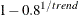), where trend is the value of the TREND= option. This produces defaults of WEIGHT=0.2 for TREND=1, WEIGHT=0.10557 for TREND=2, and WEIGHT=0.07168 for TREND=3.

The ESM procedure and the Time Series Forecasting System provide for generating forecast models that use Winters Method and enable you to specify or optimize the weights. (See Chapter 14: The ESM Procedure, and Chapter 46: Getting Started with Time Series Forecasting, for details.)

Confidence Limits

A method for calculating exact forecast confidence limits for the WINTERS method is not available. Therefore, the approach taken in PROC FORECAST is to assume that the true seasonal factors have small variability about a set of fixed seasonal factors and that the remaining variation of the series is small relative to the mean level of the series. The equations are written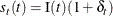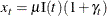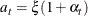whereis the mean level and I(t ) are the fixed seasonal factors. Assuming that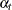and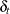are small, the forecast equations can be linearized and only first-order terms inandkept. In terms of forecasts for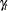, this linearized system is equivalent to a seasonal ARIMA model. Confidence limits forare based on this ARIMA model and converted into confidence limits forusingas estimates of I(t ).

The exponential smoothing confidence limits are based on an approximation to a weighted regression model, whereas the preceding Winters confidence limits are based on an approximation to an ARIMA model. You can use METHOD=WINTERS without the SEASONS= option to do exponential smoothing and get confidence limits for the EXPO forecasts based on the ARIMA model approximation. These are generally more pessimistic than the weighted regression confidence limits produced by METHOD=EXPO.

The ADDWINTERS method is like the WINTERS method except that the seasonal parameters are added to the trend instead of multiplied with the trend. The default TREND=2 model is as follows: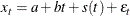The WINTERS method for updating equation and confidence limits calculations described in the preceding section are modified accordingly for the additive version.

Holt Two-Parameter Exponential Smoothing

If the seasonal factors are omitted (that is, if the SEASONS= option is not specified), the WINTERS (and ADDWINTERS) method reduces to the Holt two-parameter version of exponential smoothing. Thus, the WINTERS method is often referred to as the Holt-Winters method.

Double exponential smoothing is a special case of the Holt two-parameter smoother. The double exponential smoothing results can be duplicated with METHOD=WINTERS by omitting the SEASONS= option and appropriately setting the WEIGHT= option. Letting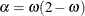and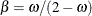, the following statements produce the same forecasts:

        proc forecast method=expo trend=2 weight=…;

        proc forecast method=winters trend=2 weight=(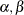) …;

Although the forecasts are the same, the confidence limits are computed differently.

Choice of Weights for EXPO, WINTERS, and ADDWINTERS Methods

For the EXPO, WINTERS, and ADDWINTERS methods, properly chosen smoothing weights are of critical importance in generating reasonable results. There are several factors to consider in choosing the weights.

The noisier the data, the lower should be the weight given to the most recent observation. Another factor to consider is how quickly the mean of the time series is changing. If the mean of the series is changing rapidly, relatively more weight should be given to the most recent observation. The more stable the series over time, the lower should be the weight given to the most recent observation.

Note that the smoothing weights should be set separately for each series; weights that produce good results for one series might be poor for another series. Since PROC FORECAST does not have a feature to use different weights for different series, when forecasting multiple series with the EXPO, WINTERS, or ADDWINTERS method it might be desirable to use different PROC FORECAST steps with different WEIGHT= options.

For the Winters method, many combinations of weight values might produce unstable noninvertible models, even though all three weights are between 0 and 1. When the model is noninvertible, the forecasts depend strongly on values in the distant past, and predictions are determined largely by the starting values. Unstable models usually produce poor forecasts. The Winters model can be unstable even if the weights are optimally chosen to minimize the in-sample MSE. See Archibald (1990) for a detailed discussion of the unstable region of the parameter space of the Winters model.

Optimal weights and forecasts for exponential smoothing models can be computed by using the ESM and ARIMA procedures and by the Time Series Forecasting System .

Starting Values for EXPO, WINTERS, and ADDWINTERS Methods

The exponential smoothing method requires starting values for the smoothed values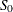,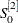, and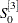. The Winters and additive Winters methods require starting values for the trend coefficients and seasonal factors.

By default, starting values for the trend parameters are computed by a time trend regression over the first few observations for the series. Alternatively, you can specify the starting value for the trend parameters with the ASTART=, BSTART=, and CSTART= options.

The number of observations used in the time trend regression for starting values depends on the NSTART= option. For METHOD=EXPO, NSTART= beginning values of the series are used, and the coefficients of the time trend regression are then used to form the initial smoothed values,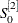, and.

For METHOD=WINTERS or METHOD=ADDWINTERS, n complete seasonal cycles are used to compute starting values for the trend parameter, where n is the value of the NSTART= option. For example, for monthly data the seasonal cycle is one year, so NSTART=2 specifies that the first 24 observations at the beginning of each series are used for the time trend regression used to calculate starting values.

The starting values for the seasonal factors for the WINTERS and ADDWINTERS methods are computed from seasonal averages over the first few complete seasonal cycles at the beginning of the series. The number of seasonal cycles averaged to compute starting seasonal factors is controlled by the NSSTART= option. For example, for monthly data with SEASONS=12 or SEASONS=MONTH, the first n January values are averaged to get the starting value for the January seasonal parameter, where n is the value of the NSSTART= option.

The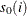seasonal parameters are set to the ratio (for WINTERS) or difference (for ADDWINTERS) of the mean for the season to the overall mean for the observations used to compute seasonal starting values.

For example, if METHOD=WINTERS, INTERVAL=DAY, SEASON=(MONTH DAY), and NSTART=2 (the default), the initial seasonal parameter for January is the ratio of the mean value over days in the first two Januaries after the start of the series (that is, after the first nonmissing value) to the mean value for all days read for initialization of the seasonal factors. Likewise, the initial factor for Sundays is the ratio of the mean value for Sundays to the mean of all days read.

For the ASTART=, BSTART=, and CSTART= options, the values specified are associated with the variables in the VAR statement in the order in which the variables are listed (the first value with the first variable, the second value with the second variable, and so on). If there are fewer values than variables, default starting values are used for the later variables. If there are more values than variables, the extra values are ignored.